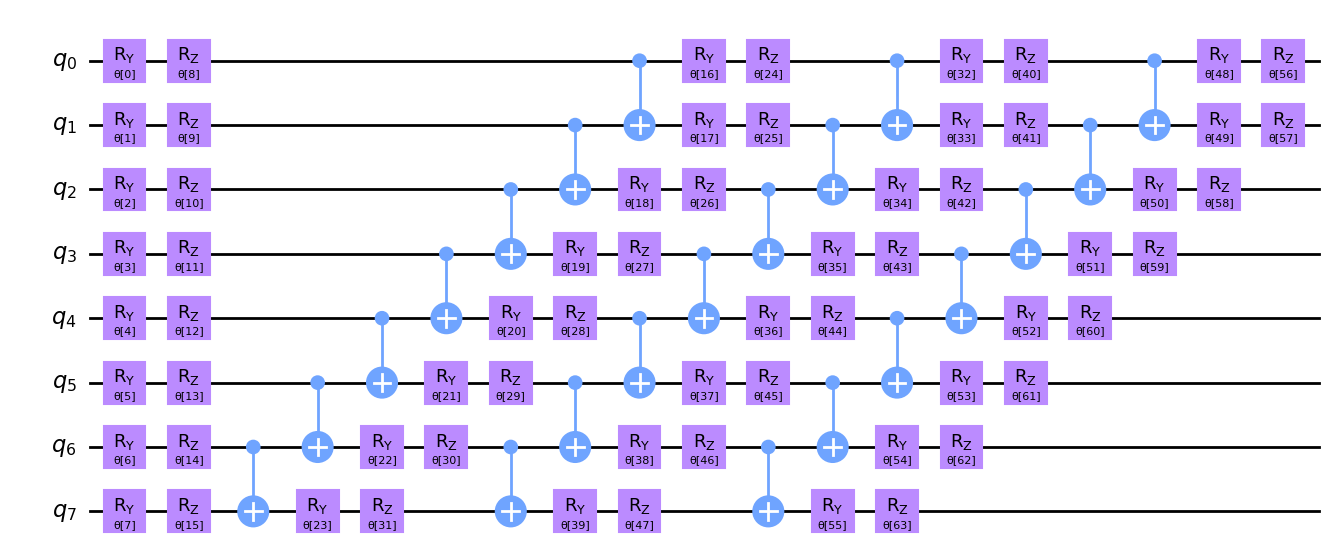# Exact and noisy simulation with Qiskit Aer primitives

Exact simulation with Qiskit primitives demonstrates how to use the reference primitives included with Qiskit to perform exact simulation of quantum circuits. Currently existing quantum processors suffer from errors, or noise, so the results of an exact simulation do not necessarily reflect the results you would expect when running circuits on real hardware. While the reference primitives in Qiskit do not support modeling noise, Qiskit Aer (opens in a new tab) includes implementations of the primitives that do support modeling noise. Qiskit Aer is a high-performance quantum circuit simulator that you can use in place of the reference primitives for better performance and more features. It is part of the Qiskit Ecosystem (opens in a new tab). In this article, we demonstrate the use of Qiskit Aer primitives for exact and noisy simulation.

Let's create an example circuit on 8 qubits.

 :
from qiskit.circuit.library import EfficientSU2

n_qubits = 8
circuit = EfficientSU2(n_qubits)
circuit.decompose().draw("mpl")

Output:This circuit contains parameters to represent the rotation angles for $R_y$ and $R_z$ gates. When simulating this circuit, we need to specify explicit values for these parameters. In the next cell, we specify some values for these parameters and use the Estimator (opens in a new tab) primitive from Qiskit Aer to compute the exact expectation value of the observable $ZZ \cdots Z$.

Note

Setting approximation=True when initializing the Estimator tells Qiskit Aer to approximate the effect of sampling error rather than actually perform sampling. This makes the simulation much more efficient, and also allows us to calculate the exact expectation value, free of sampling error. After Qiskit Aer 0.14, this will be the default behavior, so we won't need to specify this argument.

 :
from qiskit.quantum_info import SparsePauliOp
from qiskit_aer.primitives import Estimator

observable = SparsePauliOp("Z" * n_qubits)
params = [0.1] * circuit.num_parameters

exact_estimator = Estimator(approximation=True)
job = exact_estimator.run(circuit, observable, params)
exact_value = job.result().values
exact_value

Output:

/tmp/ipykernel_64852/3684797947.py:8: DeprecationWarning: qiskit_aer.primitives.estimator.Estimator.__init__()'s argument approximation is deprecated as of qiskit-aer 0.13. It will be removed no earlier than 3 months after the release date. approximation=True will be default in the future.
exact_estimator = Estimator(approximation=True)

0.8870140234256602


Now, let's initialize a noise model that includes depolarizing error of 2% on every CX gate. In practice, the error arising from the two-qubit gates, which are CX gates here, are the dominant source of error when running a circuit. See Building noise models for an overview of constructing noise models in Qiskit Aer.

In the next cell, we construct an Estimator that incorporates this noise model and use it to compute the expectation value of the observable.

 :
from qiskit_aer.noise import NoiseModel, depolarizing_error

noise_model = NoiseModel()
cx_depolarizing_prob = 0.02
depolarizing_error(cx_depolarizing_prob, 2), ["cx"]
)

noisy_estimator = Estimator(
backend_options={"noise_model": noise_model}, approximation=True
)
job = noisy_estimator.run(circuit, observable, params)
noisy_value = job.result().values
noisy_value

Output:

/tmp/ipykernel_64852/2637453528.py:9: DeprecationWarning: qiskit_aer.primitives.estimator.Estimator.__init__()'s argument approximation is deprecated as of qiskit-aer 0.13. It will be removed no earlier than 3 months after the release date. approximation=True will be default in the future.
noisy_estimator = Estimator(

0.7247404214143529


As you can see, the expectation value in the presence of the noise is quite far from the correct value. In practice, you can employ a variety of error mitigation techniques to counter the effects of the noise, but a discussion of these techniques is outside the scope of this article.

To get a very rough sense of how the noise affects the final result, consider our noise model, which adds a depolarizing error of 2% to each CX gate. Depolarizing error with probability $p$ is defined as a quantum channel $E$ that has the following action on a density matrix $\rho$:

$E(\rho) = (1 - p) \rho + p\frac{I}{2^n}$

where $n$ is the number of qubits, in this case, 2. That is, with probability $p$, the state is replaced with the completely mixed state, and the state is preserved with probability $1 - p$. After $m$ applications of the depolarizing channel, the probability of the state being preserved would be $(1 - p)^m$. Therefore, we expect the probability of retaining the correct state at the end of the simulation to go down exponentially with the number of CX gates in our circuit.

Let's count the number of CX gates in our circuit and compute $(1 - p)^m$. Because our circuit uses the EfficientSU2 class, we'll need to call decompose once to decompose it into CX gates. We call count_ops to get a dictionary that maps gate names to counts, and retrieve the entry for the CX gate.

 :
cx_count = circuit.decompose().count_ops()["cx"]
(1 - cx_depolarizing_prob) ** cx_count

Output:

0.6542558123199923


This value, 65%, gives a rough estimate of the probability that our final state is correct. It is a conservative estimate because it does not take into account the initial state of the simulation. To get a more concrete estimate of how much our final state deviates from the correct state, let's use the Sampler (opens in a new tab) primitive to estimate the final measurement probability distributions with and without noise, and then compute the fidelity between these distributions. When running the Sampler, we pass shots=None to request a final distribution that does not include random sampling error.

 :
import math
from qiskit.result import ProbDistribution
from qiskit_aer.primitives import Sampler

measured_circuit = circuit.copy()
measured_circuit.measure_all()

# Get exact probability distribution
exact_sampler = Sampler()
job = exact_sampler.run(measured_circuit, params, shots=None)
exact_quasis = job.result().quasi_dists
exact_probs = exact_quasis.nearest_probability_distribution()

# Get noisy probability distribution
noisy_sampler = Sampler(backend_options={"noise_model": noise_model})
job = noisy_sampler.run(measured_circuit, params, shots=None)
noisy_quasis = job.result().quasi_dists
noisy_probs = noisy_quasis.nearest_probability_distribution()

# Compute fidelity
def fidelity(dist1: ProbDistribution, dist2: ProbDistribution) -> float:
result = 0
for bitstring in dist1 | dist2:
prob1 = dist1.get(bitstring, 0)
prob2 = dist2.get(bitstring, 0)
result += math.sqrt(prob1 * prob2)
return result**2

fidelity(exact_probs, noisy_probs)

Output:

0.8917750028756636